$$\newcommand{\id}{\mathrm{id}}$$ $$\newcommand{\Span}{\mathrm{span}}$$ $$\newcommand{\kernel}{\mathrm{null}\,}$$ $$\newcommand{\range}{\mathrm{range}\,}$$ $$\newcommand{\RealPart}{\mathrm{Re}}$$ $$\newcommand{\ImaginaryPart}{\mathrm{Im}}$$ $$\newcommand{\Argument}{\mathrm{Arg}}$$ $$\newcommand{\norm}{\| #1 \|}$$ $$\newcommand{\inner}{\langle #1, #2 \rangle}$$ $$\newcommand{\Span}{\mathrm{span}}$$

# 2.3: Solve Equations with Variables and Constants on Both Sides

•• OpenStax
• OpenStax
$$\newcommand{\vecs}{\overset { \rightharpoonup} {\mathbf{#1}} }$$ $$\newcommand{\vecd}{\overset{-\!-\!\rightharpoonup}{\vphantom{a}\smash {#1}}}$$$$\newcommand{\id}{\mathrm{id}}$$ $$\newcommand{\Span}{\mathrm{span}}$$ $$\newcommand{\kernel}{\mathrm{null}\,}$$ $$\newcommand{\range}{\mathrm{range}\,}$$ $$\newcommand{\RealPart}{\mathrm{Re}}$$ $$\newcommand{\ImaginaryPart}{\mathrm{Im}}$$ $$\newcommand{\Argument}{\mathrm{Arg}}$$ $$\newcommand{\norm}{\| #1 \|}$$ $$\newcommand{\inner}{\langle #1, #2 \rangle}$$ $$\newcommand{\Span}{\mathrm{span}}$$ $$\newcommand{\id}{\mathrm{id}}$$ $$\newcommand{\Span}{\mathrm{span}}$$ $$\newcommand{\kernel}{\mathrm{null}\,}$$ $$\newcommand{\range}{\mathrm{range}\,}$$ $$\newcommand{\RealPart}{\mathrm{Re}}$$ $$\newcommand{\ImaginaryPart}{\mathrm{Im}}$$ $$\newcommand{\Argument}{\mathrm{Arg}}$$ $$\newcommand{\norm}{\| #1 \|}$$ $$\newcommand{\inner}{\langle #1, #2 \rangle}$$ $$\newcommand{\Span}{\mathrm{span}}$$

##### Learning Objectives

By the end of this section, you will be able to:

• Solve an equation with constants on both sides
• Solve an equation with variables on both sides
• Solve an equation with variables and constants on both sides
##### Note

Before you get started, take this readiness quiz.

1. Simplify: 4y−9+9.
If you missed this problem, review Exercise 1.10.20.

## Solve Equations with Constants on Both Sides

In all the equations we have solved so far, all the variable terms were on only one side of the equation with the constants on the other side. This does not happen all the time—so now we will learn to solve equations in which the variable terms, or constant terms, or both are on both sides of the equation.

Our strategy will involve choosing one side of the equation to be the “variable side”, and the other side of the equation to be the “constant side.” Then, we will use the Subtraction and Addition Properties of Equality to get all the variable terms together on one side of the equation and the constant terms together on the other side.

By doing this, we will transform the equation that began with variables and constants on both sides into the form $$ax=b$$. We already know how to solve equations of this form by using the Division or Multiplication Properties of Equality.

##### Exercise $$\PageIndex{1}$$

Solve: $$7x+8=−13$$.

In this equation, the variable is found only on the left side. It makes sense to call the left side the “variable” side. Therefore, the right side will be the “constant” side. We will write the labels above the equation to help us remember what goes where.Since the left side is the “xx”, or variable side, the 8 is out of place. We must “undo” adding 8 by subtracting 8, and to keep the equality we must subtract 8 from both sides.Use the Subtraction Property of Equality.Simplify.Now all the variables are on the left and the constant on the right. The equation looks like those you learned to solve earlier. Use the Division Property of Equality.Simplify.Check:Let x=−3.##### Exercise $$\PageIndex{2}$$

Solve: $$3x+4=−8$$.

$$x=−4$$

##### Exercise $$\PageIndex{3}$$

Solve: $$5a+3=−37$$.

$$a=−8$$

##### Exercise $$\PageIndex{4}$$

Solve: $$8y−9=31$$.

Notice, the variable is only on the left side of the equation, so we will call this side the “variable” side, and the right side will be the “constant” side. Since the left side is the “variable” side, the 9 is out of place. It is subtracted from the 8y, so to “undo” subtraction, add 9 to both sides. Remember, whatever you do to the left, you must do to the right.Add 9 to both sides.Simplify.The variables are now on one side and the constants on the other. We continue from here as we did earlier. Divide both sides by 8.Simplify.Check: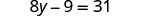Let y=5.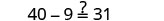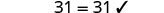##### Exercise $$\PageIndex{5}$$

Solve: $$5y−9=16$$.

$$y=5$$

##### Exercise $$\PageIndex{6}$$

Solve: $$3m−8=19$$.

$$m = 9$$

## Solve Equations with Variables and Constants on Both Sides

The next example will be the first to have variables and constants on both sides of the equation. It may take several steps to solve this equation, so we need a clear and organized strategy.

##### Exercise $$\PageIndex{7}$$

Solve: $$9x=8x−6$$.

Here the variable is on both sides, but the constants only appear on the right side, so let’s make the right side the “constant” side. Then the left side will be the “variable” side.We don’t want any x’s on the right, so subtract the 8x from both sides.Simplify.We succeeded in getting the variables on one side and the constants on the other, and have obtained the solution. Check:Let x=−6.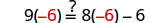##### Exercise $$\PageIndex{8}$$

Solve: $$6n=5n−10$$.

$$n = -10$$

##### Exercise $$\PageIndex{9}$$

Solve: $$-6c = -7c - 1$$

$$c = -1$$

##### Exercise $$\PageIndex{10}$$

Solve: $$5y - 9 = 8y$$

The only constant is on the left and the y’s are on both sides. Let’s leave the constant on the left and get the variables to the right.Subtract 5y from both sides.Simplify.We have the y’s on the right and the constants on the left. Divide both sides by 3.Simplify.Check:Let $$y=−3$$.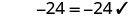##### Exercise $$\PageIndex{11}$$

Solve: $$3p−14=5p$$.

$$p = -7$$

##### Exercise $$\PageIndex{12}$$

Solve: $$8m + 9 = 5m$$

$$m = -3$$

##### Exercise $$\PageIndex{13}$$

Solve: $$12x = -x + 26$$

The only constant is on the right, so let the left side be the “variable” side.Remove the −x from the right side by adding x to both sides.Simplify.All the x’s are on the left and the constants are on the right. Divide both sides by 13.Simplify.##### Exercise $$\PageIndex{14}$$

Solve: $$12j = -4j + 32$$

$$j = 2$$

##### Exercise $$\PageIndex{15}$$

Solve: $$8h = -4h + 12$$

$$h = 1$$

## Solve Equations with Variables and Constants on Both Sides

The next example will be the first to have variables and constants on both sides of the equation. It may take several steps to solve this equation, so we need a clear and organized strategy.

##### Exercise $$\PageIndex{16}$$: How to Solve Equations with Variables and Constants on Both Sides

Solve: $$7x + 5 = 6x + 2$$##### Exercise $$\PageIndex{17}$$

Solve: $$12x+8=6x+2$$.

$$x=−1$$

##### Exercise $$\PageIndex{18}$$

Solve: $$9y+4=7y+12$$.

$$y=4$$

​​​​​​We’ll list the steps below so you can easily refer to them. But we’ll call this the ‘Beginning Strategy’ because we’ll be adding some steps later in this chapter.

##### BEGINNING STRATEGY FOR SOLVING EQUATIONS WITH VARIABLES AND CONSTANTS ON BOTH SIDES OF THE EQUATION.
1. Choose which side will be the “variable” side—the other side will be the “constant” side.
2. Collect the variable terms to the “variable” side of the equation, using the Addition or Subtraction Property of Equality.
3. Collect all the constants to the other side of the equation, using the Addition or Subtraction Property of Equality.
4. Make the coefficient of the variable equal 1, using the Multiplication or Division Property of Equality.
5. Check the solution by substituting it into the original equation.

In Step 1, a helpful approach is to make the “variable” side the side that has the variable with the larger coefficient. This usually makes the arithmetic easier.

##### Exercise $$\PageIndex{19}$$

Solve: $$8n−4=−2n+6$$.

In the first step, choose the variable side by comparing the coefficients of the variables on each side.

 Since $$8>−2$$, make the left side the “variable” side.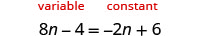We don’t want variable terms on the right side—add 2n to both sides to leave only constants on the right.Combine like terms.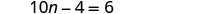We don’t want any constants on the left side, so add 4 to both sides.Simplify.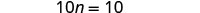The variable term is on the left and the constant term is on the right. To get the coefficient of nn to be one, divide both sides by 10.Simplify.Check:Let n=1.##### Exercise $$\PageIndex{20}$$

Solve: $$8q - 5 = -4q + 7$$

$$q = 1$$

##### Exercise $$\PageIndex{21}$$

Solve: $$7n - 3 = n + 3$$

$$n = 1$$

##### Exercise $$\PageIndex{22}$$

Solve: $$7a -3 = 13a + 7$$

In the first step, choose the variable side by comparing the coefficients of the variables on each side.

Since 13>7, make the right side the “variable” side and the left side the “constant” side.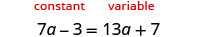Subtract 7a from both sides to remove the variable term from the left.Combine like terms.Subtract 7 from both sides to remove the constant from the right.Simplify.Divide both sides by 6 to make 1 the coefficient of a.Simplify.Check:Let $$a=−\frac{5}{3}$$.##### Exercise $$\PageIndex{23}$$

Solve: $$2a - 2 = 6a + 18$$

$$a = -5$$

##### Exercise $$\PageIndex{24}$$

Solve: $$4k -1 = 7k + 17$$

$$k = -6$$

In the last example, we could have made the left side the “variable” side, but it would have led to a negative coefficient on the variable term. (Try it!) While we could work with the negative, there is less chance of errors when working with positives. The strategy outlined above helps avoid the negatives!

To solve an equation with fractions, we just follow the steps of our strategy to get the solution!

##### Exercise $$\PageIndex{25}$$

Solve: $$\frac{4}{5}x + 6 = \frac{1}{4}x - 2$$

Since $$\frac{5}{4} > \frac{1}{4}$$, make the left side the “variable” side and the right side the “constant” side.Subtract $$\frac{1}{4}x$$ from both sides.Combine like terms.Subtract 6 from both sides.Simplify.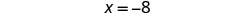Check: Let $$x = -8$$ $$\begin{array} {ccc} {\frac{5}{4}x + 6} &{=} &{\frac{1}{4}x - 2} \\ {\frac{5}{4}(-8) + 6} &{\stackrel{?}{=}} &{\frac{1}{4}(-8) - 2} \\ {-10 + 6} &{\stackrel{?}{=}} &{-2 - 2} \\ {-4} &{=} &{-4\checkmark} \end{array}$$
##### Exercise $$\PageIndex{26}$$

Solve: $$\frac{7}{8}x - 12 = -\frac{1}{8}x - 2$$

$$x = 10$$

##### Exercise $$\PageIndex{27}$$

Solve: $$\frac{7}{6}x + 11 = \frac{1}{6}y + 8$$

$$y = -3$$

We will use the same strategy to find the solution for an equation with decimals.

##### Exercise $$\PageIndex{28}$$

Solve: $$7.8x+4=5.4x−8$$.

Since $$7.8>5.4$$, make the left side the “variable” side and the right side the “constant” side.Subtract 5.4x from both sides.Combine like terms.Subtract 4 from both sides.Simplify.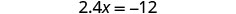Use the Division Property of Equality.Simplify.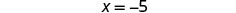Check:Let $$x=−5$$##### Exercise $$\PageIndex{29}$$

Solve: $$2.8x + 12 = -1.4x - 9$$

$$x = -5$$

##### Exercise $$\PageIndex{30}$$

Solve: $$3.6y + 8 = 1.2y - 4$$

$$y = -5$$

## Key Concepts

• Beginning Strategy for Solving an Equation with Variables and Constants on Both Sides of the Equation
1. Choose which side will be the “variable” side—the other side will be the “constant” side.
2. Collect the variable terms to the “variable” side of the equation, using the Addition or Subtraction Property of Equality.
3. Collect all the constants to the other side of the equation, using the Addition or Subtraction Property of Equality.
4. Make the coefficient of the variable equal 1, using the Multiplication or Division Property of Equality.
5. Check the solution by substituting it into the original equation.

2.3: Solve Equations with Variables and Constants on Both Sides is shared under a CC BY license and was authored, remixed, and/or curated by OpenStax.